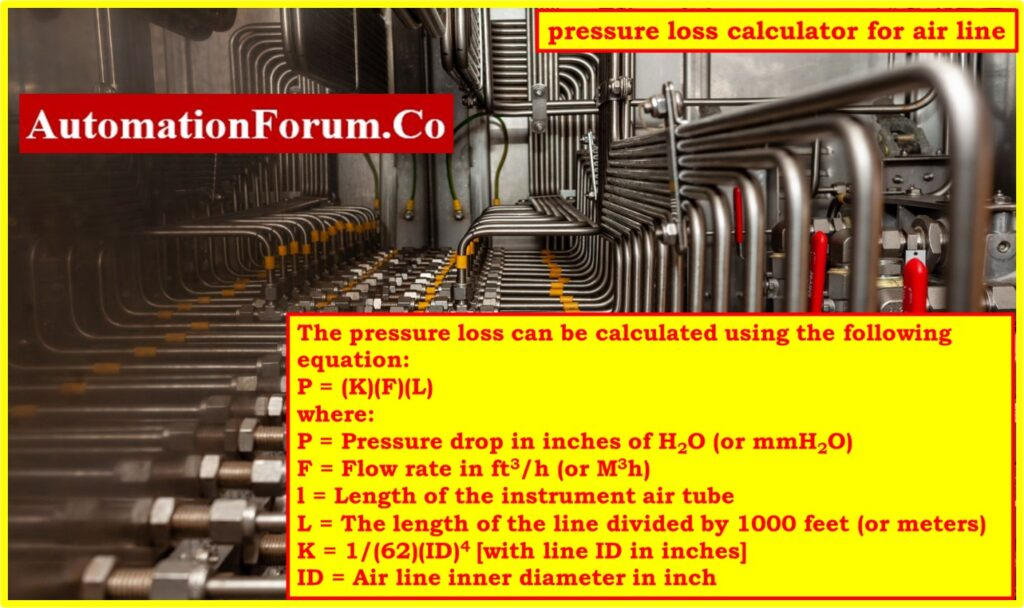# Calculator for Pressure Loss in instrument Air line• When determining the amount of pressure that is lost in the instrumentation tubing and pipes, it is important to take into account a number of different elements, including length, diameter, flow rate, fittings, and surface roughness.
When estimating the amount of pressure lost, it is best to make use of well-known equations such as the Darcy-Weisbach or Hazen-Williams equations.
• Make sure that the values for factors like flow rate, pipe diameter, and roughness are accurate.
• Take into mind the particular qualities of the tubing and pipe used in instruments, which tend to have smaller diameters and lower flow rates. Consulting engineering references, standards, or specialized software can provide more precise calculations.
• Maintaining accurate pressure readings and ensuring optimal performance in instrumentation tubing and pipe systems both need accurate estimates of the pressure loss that occur in these systems.

## Formula for pressure loss calculation

The flow rate, inside diameter of the line, and the length of the line all have an effect on the amount of pressure that is lost in the line. The pressure loss can be calculated using the following equation:

P = (K)(F)(L)

Where,

P = Pressure drop in inches of H2O (or mmH2O)

F = Flow rate in ft3/h (or M3h)

l = Length of the instrument air tube

L = Length of the line divided by 1000 feet (or meters)

ID = Air line inner diameter in inch

The line ID and the other factors are combined into a single global constant, K, which is presented in the accompanying table.

## Calculation of K value

Use the calculation below to determine the approximate value of K if the ID of the actual pipe used is not stated in the table above.

K = 1/(62)(ID)4 [with line ID in inches]

## Calculation of K value for SI units

Use the following formula to determine K if the measurements are in the SI system (line ID in mm, line length in meters, and flow in m3/h).

K in SI units = (65.9/ID)4

## Example calculation 1:

Determine pressure loss in a 25 foot length of 1/8 pipe due to an air flow of 5 scfh.

For 1/8 in pipe, K = 3.0 (from the table)

P = (K)(F)(L)

P = (3.0)(5)(25/1000)

P = 0.375 inH2O

## Example calculation 2:

Determine pressure loss in a 15 meter length of 5 mm ID pipe due to an air flow of 0.2 m3 /h.

K in SI units = (65.9/ID)4

K in SI units = (65.9/5)4 = 30176

P = (K)(F)(L)

P = (30176)(0.2)(15/1000)

P = 90.53 mmH2O

## Calculator for Pressure Loss in Air Line

The pressure loss in instrument compressed air lines can be calculated with the help of the calculator that is provided below.

Scroll to Top